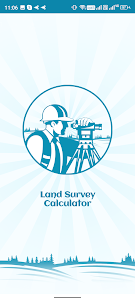# Land Survey Calculator - LSC v1.0.6

Free

### Land Survey Calculator - LSC / Screenshots### Land Survey Calculator - LSC / Description

Land Survey Calculator is the best Land survey calculator and Area calculator offline.

Land Survey Calculator you can find the Measurement of Your Land Area calculator and also find Area converter Area formula and you can also solve Algebra and it will also be shown all the explanation of your Algebra. All Calculators at one place have no need to go anywhere else to do different calculations. Land Survey Calculator includes amazing features that include…

1. Land Survey Calculator
2. Converters
3. Area calculator
4. Geometry calculator
5. Algebra Calculator

Land Survey Calculator

This calculator can evaluate the area of triangle and rectangle shapes. You have to do is add a triangle or rectangle and put two values and it will give you a calculation in a square meter. Simple and easy to use. The area of more complex shapes can be usually be obtained by breaking them down into their aggregate simple shapes and the total of their area. This calculator is especially useful for estimating land area.

Converters

Here you can convert your Area. this converter you can use everywhere anywhere. You also convert your area when you don’t have any internet access because it works offline as well. You just enter what you are what you convert it and select unit ( Cents Acre Hectare Square Meter ) what you want it and just hit submit button and the Area is completely ready.

Area Calculators

Remember the Area formula???
No?

Then you don’t have to worry about that it will also give you an Area formula You can see various of the areas in this Calculator. just choose your area that you want to convert it and it will give you a quick result.

Geometry

A handful of useful geometry calculators to help you solve your Geometry problems. Choose your Geometry area to be calculation and put value to convert it and quick result of your Geometry calculation. There are multiple Geometry shown so you don’t have to think just select it and calculate. You can find the solution of Geometry such as Cylinder Pythagoras Circular Ring Sphere Cube Rectangular box right circular cone frustum of a cone. Frustum of a cone. This Calculator is used to check homework answers practice or explore various values for deep understanding.

Algebra

Solve your Arithmetic Algebra in the Land Servey Calculator. The associative commutative and destructive Algebra properties are laws applied to addition and multiplication that always exist. The associative property states that you can re-group numbers and you will get the same answer and the commutative property states that you can move numbers and still arrive at the same answer. You can calculate three of Algebra Arithmetic associative Arithmetic commutative and Arithmetic distributive.

Arithmetic associative

The associative property comes from the word associate or group it refers to the grouping of numbers or variables in algebra. You can re-group the numbers or variables and you will always arrive at the same answer. This formula is shown in the Arithmetic associative property a(bc)=(ab)c

Arithmetic commutative

The commutative property in math comes from the words commute or move around this rule states that you can move numbers or variables in algebra around and still get the same answer. This formula is shown in the Arithmetic commutative property a+b=b+a and ab=ba

Arithmetic distributive

The distributive property lets you multiply a sum by multiplying each addend separately and then added all the products. This was a formula of the distributive property a(b+c)=ab+ac

Use Land Survey Calculator and solve your math problems easily. If you have any queries and suggestions then contact us at [email protected]